Next: Conformal Maps Up: Two-Dimensional Potential Flow Previous: Complex Velocity

# Method of Images

Let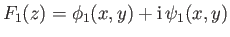and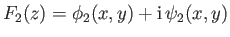be complex velocity potentials corresponding to distinct, two-dimensional, irrotational, incompressible flow patterns whose stream functions are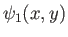and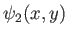, respectively. It follows that both stream functions satisfy Laplace's equation: that is,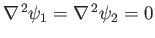. Suppose that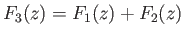. Writing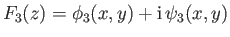, it is clear that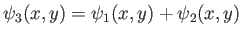. Moreover,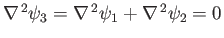, so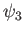also satisfies Laplace's equation. We deduce that two complex velocity potentials, corresponding to distinct, two-dimensional, irrotational, incompressible flow patterns, can be superposed to produce a third velocity potential that corresponds to another two-dimensional, irrotational, incompressible flow pattern. As described in the following, this idea can be exploited to determine the flow patterns produced by line sources and vortex filaments in the vicinity of rigid boundaries.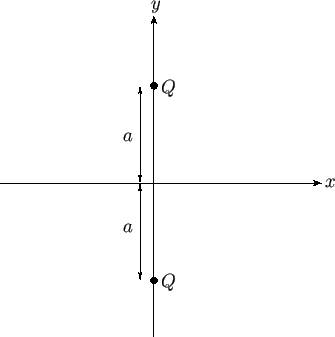As an example, consider a situation in which there are two line sources of strength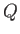located at the points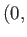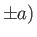. (See Figure 6.1.) The complex velocity potential of the resulting flow pattern is the sum of the complex potentials of each source taken in isolation. Hence, from Section 6.4,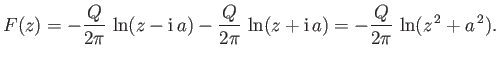(6.42)

Thus, the stream function of the flow pattern (which is the imaginary part of the complex potential) is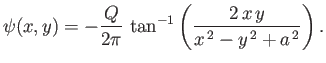(6.43)

Note that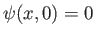, which implies that there is zero flow normal to the plane. Hence, in the region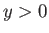, we could interpret the previous stream function as that generated by a single line source of strength, located at the point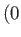,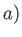, in the presence of a planar rigid boundary at. This follows because the stream function satisfies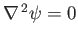everywhere in the region, has the requisite singularity (corresponding to a line source of strength) at,, and satisfies the physical boundary condition that the normal velocity be zero at the rigid boundary. Moreover, as is well-known, the solutions of Laplace's equation are unique. The streamlines of the resulting flow pattern are shown in Figure 6.2. Incidentally, we can think of the two sources in Figure 6.1 as the images'' of one another in the boundary plane. Hence, this method of calculation is usually referred to as the method of images.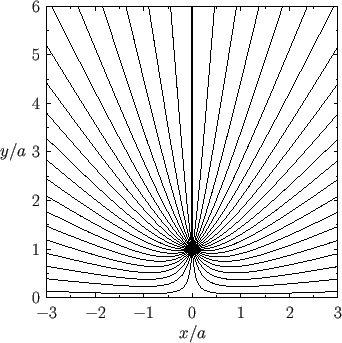The complex velocity associated with the complex velocity potential (6.42) is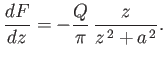(6.44)

Hence, the flow speed at the boundary is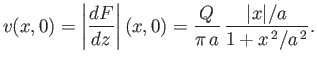(6.45)

It follows from Equation (6.41) (and the fact that the flow speed at infinity is zero) that the excess pressure on the boundary, due to the presence of the source, is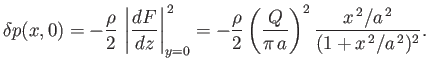(6.46)

Thus, the excess force per unit length (in the-direction) acting on the boundary in the-direction is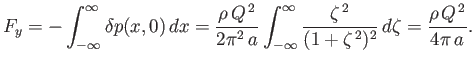(6.47)

The fact that the force is positive implies that the boundary is attracted to the source, and vice versa.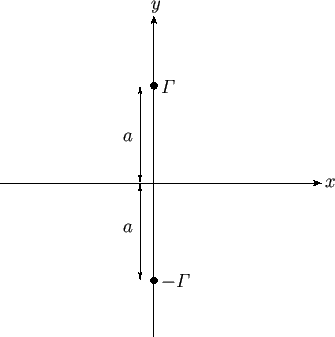As a second example, consider the situation, illustrated in Figure 6.3, in which there are two vortex filaments of intensities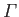and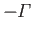situated at,. As before, the complex velocity potential of the resulting flow pattern is the sum of the complex potentials of each filament taken in isolation. Hence, from Section 6.4,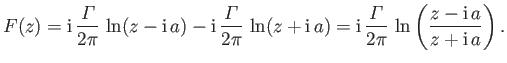(6.48)

Thus, the stream function of the flow pattern is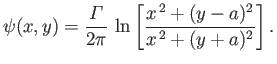(6.49)

As before,, which implies that there is zero flow normal to the plane(because the plane corresponds to a streamline). Hence, in the region, we could interpret the previous stream function as that generated by a single vortex filament of intensity, located at the point,, in the presence of a planar rigid boundary at. The streamlines of the resulting flow pattern are shown in Figure 6.4. We conclude that a vortex filament reverses its sense of rotation (i.e.,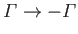) when reflected'' in a boundary plane.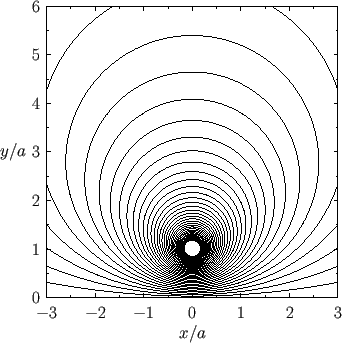As a final example, consider the situation, illustrated in Figure 6.5, in which there is an impenetrable circular cylinder of radius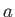, centered on the origin, and a line source of strengthlocated at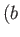,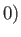, where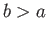. Consider the so-called analog problem, also illustrated in Figure 6.5, in which the cylinder is replaced by a source of strength, located at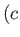,, where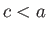, and a source of strength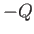, located at the origin. We can think of these two sources as the images'' of the external source in the cylinder. Moreover, given that the solutions of Laplace's equation are unique, if the analog problem can be adjusted in such a manner that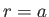is a streamline then the flow in the regionwill become identical to that in the actual problem. The complex velocity potential in the analog problem is simply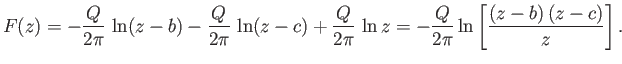(6.50)

Hence, writing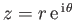, the corresponding stream function takes the form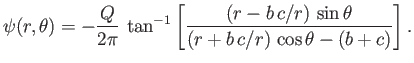(6.51)

We require the surface of the cylinder,, to be a streamline: that is,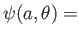constant. This is easily achieved by setting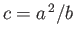. Thus, the stream function becomes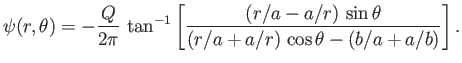(6.52)

The corresponding streamlines in the region external to the cylinder are illustrated in Figure 6.6.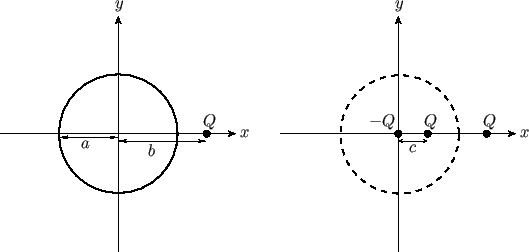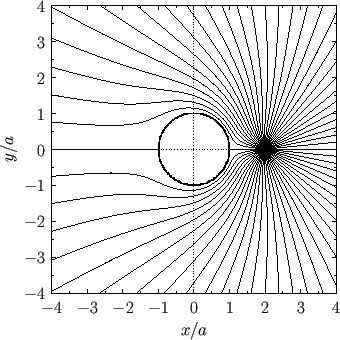Next: Conformal Maps Up: Two-Dimensional Potential Flow Previous: Complex Velocity
Richard Fitzpatrick 2016-03-31